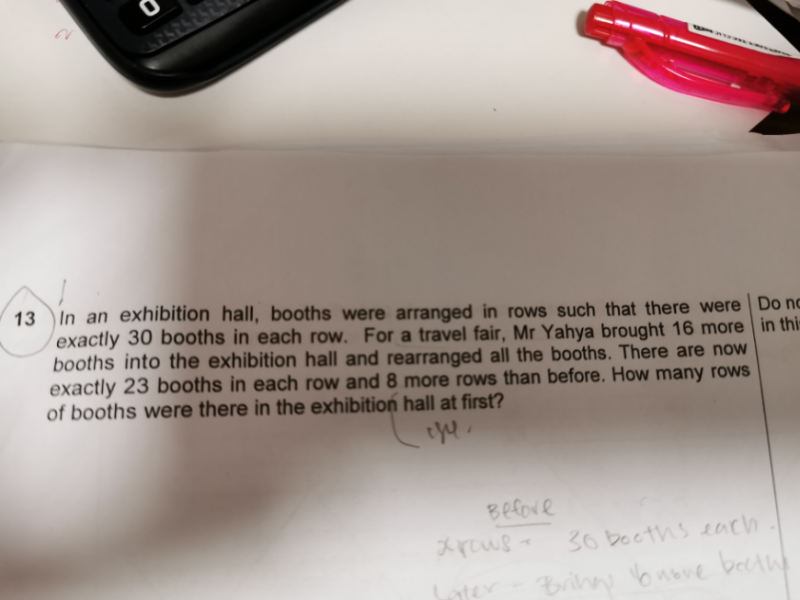# QuestionThanks 😊

The difficulty here is to adapt words into an equation.

Let’s say x=the number of rows at the beginning
Therefore we have:

Number total of booths at the beginning = 30x

Number total of booths after Mr. Yahya brought more = 30x + 16

Then we know according to the wording:

30x+16=23(x+8)  // (x+8) equals to the new number of rows

Then it’s just a simple equation to solve

30x = 23x + 184 – 16

7x= 168

x= 24

At first, there were 24 rows in the exhibition. 🙂

0 Replies 0 Likes ✔Accepted Answer

If not using algebra, but to explain below if the child can understand the scenario:

there are now 8 more rows of 23 booths = 8 x 23 = 184 booths.

Of which 16 booths are the “extras brought  in” and

And the other booths (184-16=168) came from the “pulled-out 7 booths per row”.  (30-23=7)

168/7 = 24 (original) rows at first.

0 Replies 0 Likes

Thank you 😊

0 Replies 0 Likes